# 資料結構與演算法—棧詳解

### 什麼是棧### 陣列實現

``````private T data[];
private int top;
public seqStack() {
data=(T[]) new Object;
top=-1;
}
public seqStack(int maxsize)
{
data=(T[]) new Object[maxsize];
top=-1;
}
``````

push插入

• 如果top<陣列長度-1。入棧,`top++;a[top]=value;`
• 如果top==陣列長度-1；棧滿。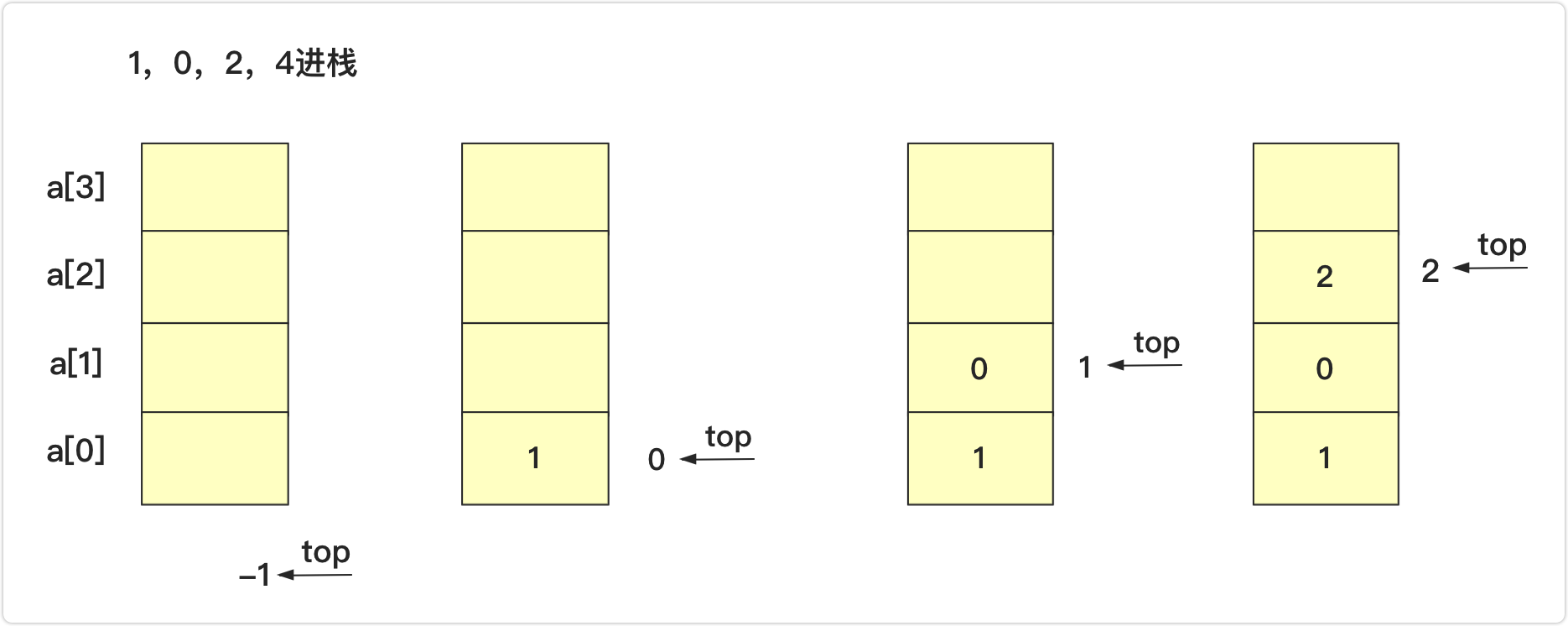pop彈出並返回首位

• 如果top>=0,棧不為空，可以彈出。`return data[top--];`
• 如下圖，本來棧為1,2,3,4,5,6（棧頂）,執行pop操作,top變為3的位置並且返回4；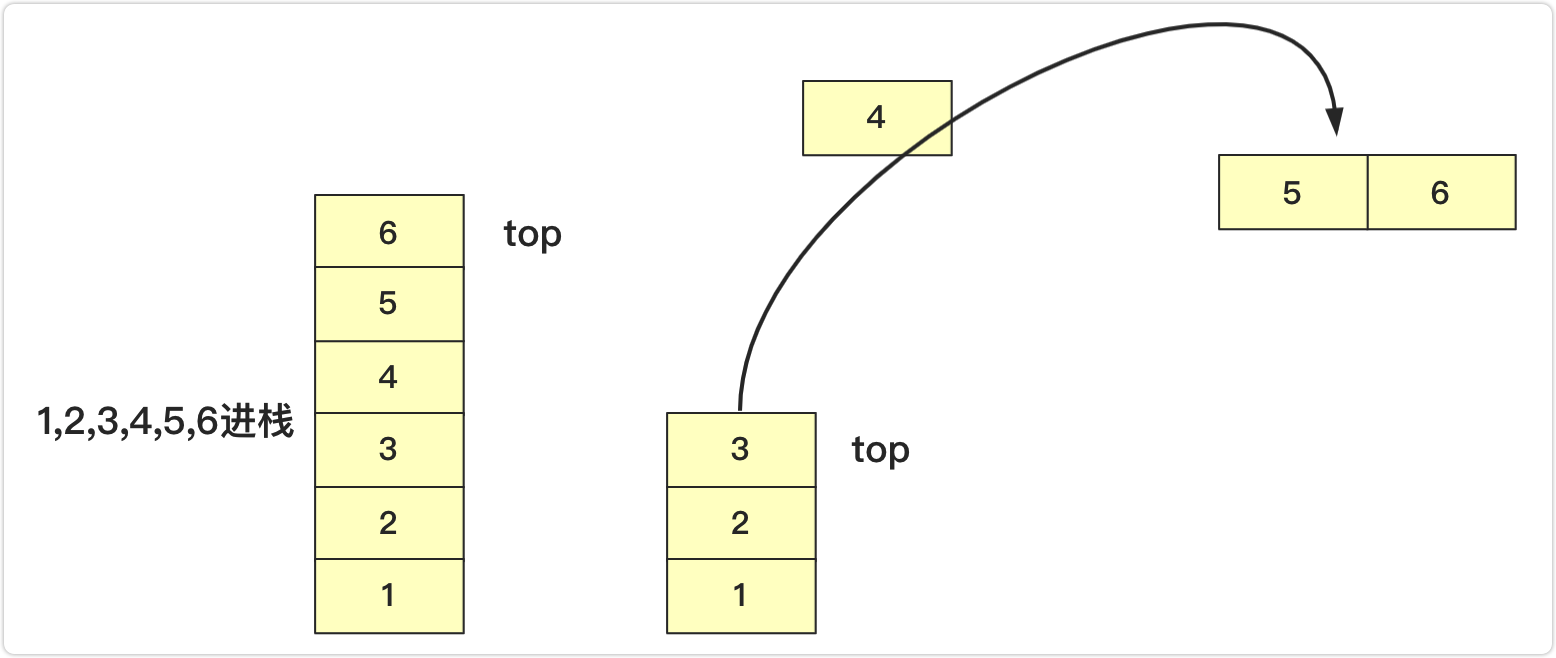``````package 隊棧;

public class seqStack<T> {

private T data[];
private int top;
public seqStack() {
data=(T[]) new Object;
top=-1;
}
public seqStack(int maxsize)
{
data=(T[]) new Object[maxsize];
top=-1;
}
boolean isEmpty()
{
}
int length()
{
}

boolean push(T value) throws Exception//壓入棧
{
if(top+1>data.length-1)
{
throw new Exception("棧已滿");
}
else {
data[++top]=value;
return true;
}
}
T peek() throws Exception//返回棧頂元素不移除
{
if(!isEmpty())
{
return data[top];
}
else {
throw new Exception("棧為空");
}
}
T pop() throws Exception
{
if(isEmpty())
{
throw new Exception("棧為空");
}
else {
return data[top--];
}
}
public String toString()
{
if(top==-1)
{
return "";
}
else {
String va="";
for(int i=top;i>=0;i--)
{
va+=data[i]+"  ";
}
return va;
}
}
}

``````

### 連結串列實現

• 像陣列那樣在尾部插入刪除。大家都知道連結串列效率低在查詢，而查詢到尾部效率很低，就算用了尾指標，可以解決尾部插入效率，但是依然無法解決刪除效率(刪除需要找到前驅節點)，還需要雙向連結串列。前面雖然詳細介紹過雙向連結串列，但是這樣未免太複雜
• 所以我們採用帶頭節點的單鏈表在頭部插入刪除，把頭當成棧頂，插入直接在頭節點後插入，刪除也直接刪除頭節點後第一個節點即可，這樣就可以完美的滿足棧的需求。

``````static class node<T>
{
T data;
node next;
public node() {
}
public node(T value)
{
this.data=value;
}
}
``````

``````public class lisStack <T>{
int length;
public lisStack() {
length=0;
}
//其他方法
}
``````

push插入

• 空連結串列入棧`head.next=team;`
• 非空入棧`team.next=head.next;head.next=team;`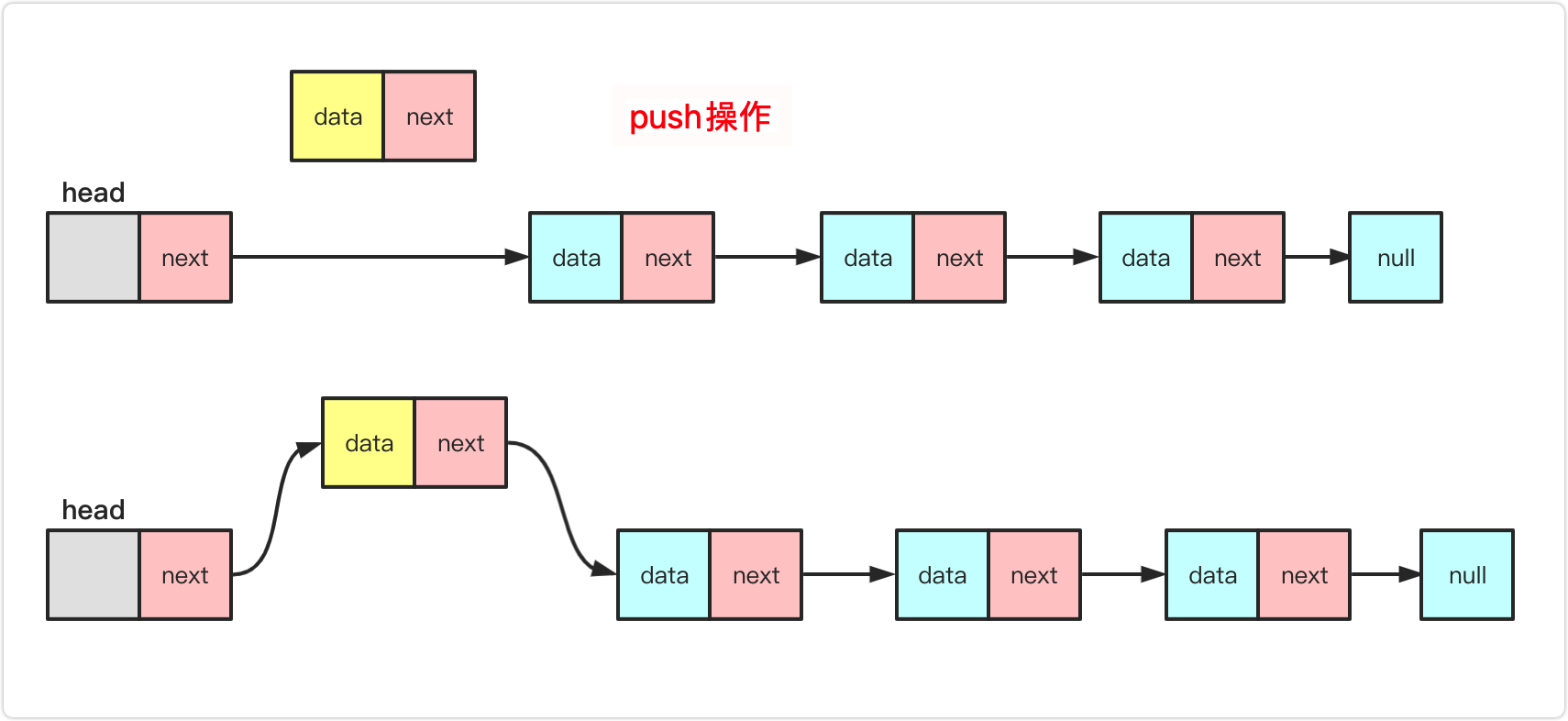pop彈出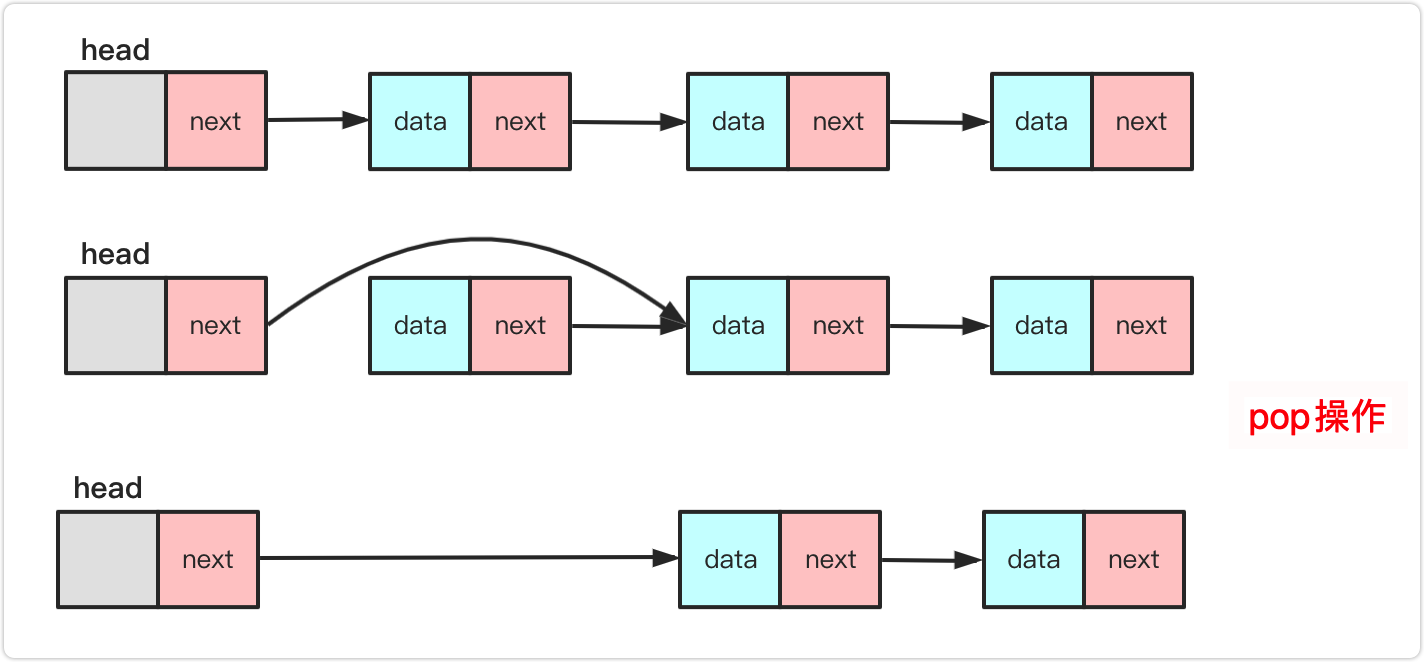``````package 隊棧;

public class lisStack <T>{
static class node<T>
{
T data;
node next;
public node() {
}
public node(T value)
{
this.data=value;
}
}
int length;
public lisStack() {
length=0;
}
boolean isEmpty()
{
}
int length()
{
return length;
}
public void push(T value) {//近棧
node<T> team=new node<T>(value);
if(length==0)
{
}
else {
length++;
}
public T peek() throws Exception {
if(length==0) {throw new Exception("連結串列為空");}
else {//刪除
}
}
public T pop() throws Exception {//出棧
if(length==0) {throw new Exception("連結串列為空");}
else {//刪除
length--;
return value;
}
}
public String toString(){
if(length==0) {return "";}
else {
String va="";
while(team!=null)
{
va+=team.data+" ";
team=team.next;
}
return va;
}
}
}
``````

### 棧能這麼玩

• `(({[(`下一個`)`消掉成`(({[`
• `(({[(`下一個`)`消掉成`(({[`
• `(({[`下一個`]`消掉成`(({`
• `(({`下一個`}`消掉成`((`
• `((`下一個`)`消掉成`(`
• `(`下一個`)`消掉成` ` 這樣就滿足題意

``````public boolean isValid(String s) {
Stack<Character>stack=new Stack<Character>();
for(int i=0;i<s.length();i++)
{
char te=s.charAt(i);
if(te==']')
{
if(!stack.isEmpty()&&stack.pop()=='[')
continue;
else {
return false;
}
}
else if(te=='}')
{
if(!stack.isEmpty()&&stack.pop()=='{')
continue;
else {
return false;
}
}
else if(te==')')
{
if(!stack.isEmpty()&&stack.pop()=='(')
continue;
else {
return false;
}
}
else
stack.push(te);
}
return stack.isEmpty();
}
``````

``````public boolean isValid(String s) {
char a[]=new char[s.length()];
int index=-1;
for(int i=0;i<s.length();i++)
{
char te=s.charAt(i);
if(te==']')
{
if(index>=0&&a[index]=='[')
index--;
else {
return false;
}
}
else if(te=='}')
{
if(index>=0&&a[index]=='{')
index--;
else {
return false;
}
}
else if(te==')')
{
if(index>=0&&a[index]=='(')
index--;
else {
return false;
}
}
else
a[++index]=te;
}
return index==-1;
}
``````

``````public  int longestValidParentheses(String s) {
char str[]=s.toCharArray();//字元陣列
int max=0;
for(int i=0;i<str.length-1;i++)
{
int index=-1;
if(max>=str.length-i)
break;
for(int j=i;j<str.length;j++)
{
if(str[j]=='(')
index++;
else {
if(index<0)
{
i=j;
break;
}
else {
index--;
}
}
if(index==-1&&(j-i+1>max))
{
max=j-i+1;
}
}
}
return max;
}
``````

• `( ) ) ` `( ) ( ( ) ( ) )` 最大為後面部分(空格分開)
• `( ) ( ) ` `( ( ( )` 最大為前面部分
• `( ( ( ( ( ` `( ) ( ) ( ) ( )` 最大為後面部分

• 一種是當前已經超過左括號前面已經不可能連續了。例如`( ) ) ( )`第三個括號出現已經使得整個串串不可能連續，最大要麼在其左面要麼再其右面。 你可以理解其為一種清零初始機制。
• 另一種情況`)`就是目標棧中存在`(`可與其進行匹配。匹配之後要疊加到消除後平級的數量上，並且判斷是否是最大值。(下面會解釋)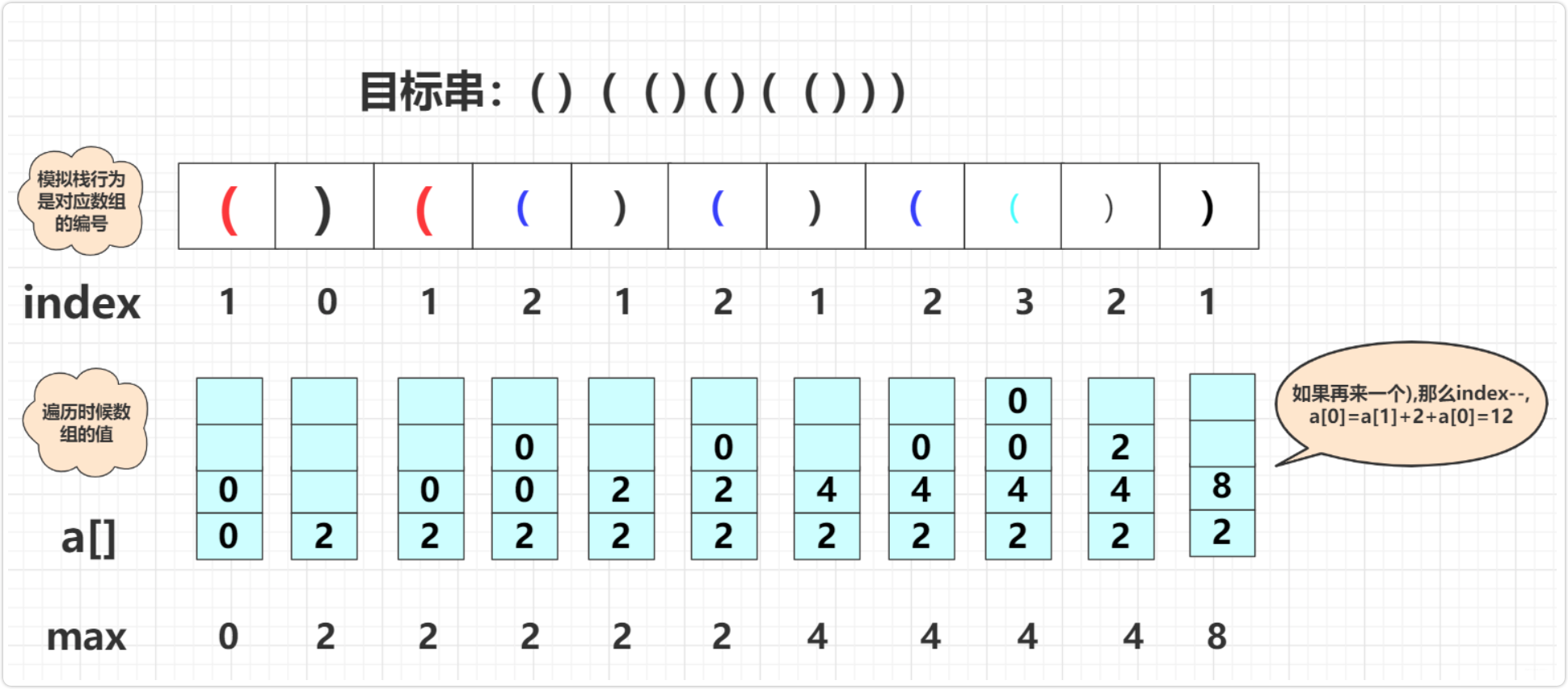`````` public static int longestValidParentheses(String s) {
int max=0;
int value[]=new int[s.length()+1];
int index=0;
for(int i=0;i<s.length();i++)
{
if(s.charAt(i)=='(')
{
index++;
value[index]=0;
}
else {//")"
if(index==0)
{
value=0;
}
else {
value[index-1]+=value[index--]+2;//疊加
if(value[index]>max)//更新
max=value[index];
}
}
}
return max;
}
``````

``````public int longestValidParentheses(String s) {
int maxans = 0;
Stack<Integer> stack = new Stack<>();
stack.push(-1);
for (int i = 0; i < s.length(); i++) {
if (s.charAt(i) == '(') {//(將當前的
stack.push(i);
} else {
stack.pop();
if (stack.empty()) {
stack.push(i);
} else {//i-stack.peek就是i是出現的總個數 peek是還沒匹配的個數
maxans = Math.max(maxans, i - stack.peek());
}
}
}
return maxans;
}
``````

「其他文章」